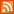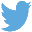Bucaro TecHelpHTTPS Encryption not required because no account numbers orpersonal information is ever requested or accepted by this siteCustom Search# Working With Numbers in PHP

In PHP there are two main types of numbers, integers (numbers without a decimal point), and floats (numbers with a decimal point). You can declare an initialize a number as shown below.

```\$amount = 62;
```

Sometimes, when you first declare a number, you may not know its value. You can declare the variable, and initialize it later in the program flow as shown below.

```\$amount;
.
.
.
\$amount = 62;
```

However, until you initialize the variable, PHP has no idea what data type you will be storing in it, so it sets its value to NULL. If you don't know a numbers value when you first declare it, it's better to set it to a default value like 0;

```\$amount = 0;
.
.
.
\$amount = 62;
```

Unlike string variables, numbers are not placed within quotes. Digits within quotes would create a string variable. If you want to convert digits within quotes to a number, you cast it to an integer or float variable type. Shown below, digits in a string variable are cast to an integer before being used in a mathematical operation.

```<?php

\$a = "23";
\$b = 32;
\$c = \$b + (integer) \$a;
print \$c;

?>
```

In many cases you don't need to cast a digits in a string to an integer or float because PHP will automatically convert a variable's type based upon the the context in which it's used. Shown below is an example of PHP automatic variable type conversion.

```<?php

\$a = "23";
\$b = 32;
\$c = \$b + \$a;
print \$c;

?>
```

Arithmetic Operators

PHP includes the standard Arithmetic Operators.

 Operator Meaning + addition - subtraction or negation * multiplication / division % modulus ++ increment -- decrement

The modulus operator performs division, except instead of returning the quotient, it returns the remainder. An example of modulus division is shown below.

```\$a = 50 % 6;
print \$a;
```

The result of execution of the lines of code above would be a print of 2.

The increment operator increases the value by 1. the decrement operator decreases the value by one. An example of decrement is shown below.

```\$a = 50;
\$a--;
print \$a;
```

The result of execution of the lines of code above would be a print of 49.

When using arithmetic operators, you can also use the shortcut method shown below.

```\$a = 26;
\$b = 14;
\$a += \$b;
print \$a;
```

 .menubtn { margin-top:10px; margin-left:50px; width:220px; opacity:1; border-style:solid; border-color:#009000; background-color:#66ff66; transition-property: opacity; transition-duration: 1s; transition-timing-function: ease-out; } .menubtn:hover { opacity:1; background-color:#e5ff23; } Menu - More SQL (Structured Query Language)RSS FeedFollow @Stephen Bucaro[Site User Agreement] [Privacy Policy] [Site map] [Search This Site] [Contact Form]
Copyright©2001-2021 Bucaro TecHelp 13771 N Fountain Hills Blvd Suite 114-248 Fountain Hills, AZ 85268